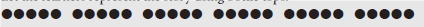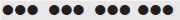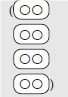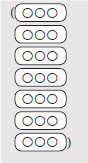# Lesson Notes By Weeks and Term - Primary 2

Multiplication

TERM: 2nd Term

WEEK: 11

CLASS: Primary 2

AGE: 7 years

DURATION: 5 periods of 40 minutes each

DATE:

SUBJECT: Mathematics

TOPIC: Multiplication

SPECIFIC OBJECTIVES: At the end of the lesson, the pupils should be able to

1. Solve problems using repeated addition.
2. Recognise the relationship between repeated addition and multiplication.
3. Solve problems involving repeated addition and multiplication.
4. Write multiplication number sentences.

INSTRUCTIONAL TECHNIQUES: Explanation, question and answer, demonstration, practical

INSTRUCTIONAL MATERIALS: Bottle tops

PRESENTATION

TEACHER’S ACTIVITY

PUPIL’S ACTIVITY

STEP 1

MENTAL MATHS

The teacher begins the lesson with some mental calculations

The teacher

- Asks the learners to stand up.

- Explains to them that they will be counting forwards in fives up

to 50. Once they have counted they are to sit down. Let the learners begin to count. When they get to 20 stop the counting.

- Asks: How many fives in 20? Let the learners continue to count.

- Repeats the question ‘how many fives’ when you get to 30, 45 and 50. - - Lets each learner have a chance to answer. You may need to repeat the counting exercise.

Pupils respond and participate

STEP 2

CONCEPT

DEVELOPMENT

The teacher

• Invites 7 children to come to the front of the class.

• Asks: How many eyes do 7 children have? (14) How did you get the answer? (7 groups of 2 is 14.) Write the following on the board: 2 + 2 + 2 + 2 +2 +2 + 2 = 14, 14 eyes.

• Solves the following with the learners using repeated addition:

How many wheels do 5 cars have? (4 + 4 + 4 + 4 + 4 = 20, 20 wheels)

How many fingers are there on 3 hands? (5 + 5 + 5 = 15, 15 fingers)

How many wheels are there on 4 tricycles? (3 + 3 + 3 + 3 = 12, 12 wheels)

CLASS ACTIVITY

The teacher

Uses bottle tops for this activity.

Counts out 12 bottle tops. Ask the learners to group the bottle tops in 2s.

Asks: How many groups of 2 are there? (6). Write on the board 2 + 2 + 2 + 2 + 2 + 2 = 12.

Lets learners write the number sentence in their classwork books.

Repeats this activity using different group sizes:

How many groups of 3 in 12? (4)

How many groups of 4 in 12? (3)

Asks: What is the relationship between 4 groups of 3 and 3 groups of 4? (They are both 12. Use bottle tops to demonstrate this.).

Lets learners write the number sentences (repeated addition) of 4 groups of 3 and 3 groups of 4 in their classwork books. (3 + 3 + 3 + 3 = 12, 4 + 4 + 4 = 12)

Writes these number sentences on the board for correction.

Discusses the relationship between 6 groups of 2 is 12, 4 groups of 3 is 12 and 3 groups of 4 is 12, using bottle tops.

Pupils pay attention and participate

STEP 3

CLASS-WORK

NOTE: In this activity learners are expected to find the answers by counting forwards in 2s,

3s, 4s, and 5s.

Complete the table.

 What is? Repeated addition Answer 1. 5 groups of 2 2 + 2 + 2 + 2 + 2 10 2. 3 groups of 3 3 + 3 + 3 3. 6 groups of 4 6 + 6 + 6 + 6 4. 3 groups of 5 5. 10 groups of 2 6. 7 groups of 3 7. 6 groups of 5 8. 8 groups of 4 9. 9 groups of 3 10. 10 groups of 5

Pupils attempt their class work

STEP 4

HOME-WORK

Complete the table.

 What is? Repeated addition Answer 1. 6 groups of 2 2. 7 groups of 3 3. 5 groups of 4

The pupils writes it in their homework book

STEP 5

SUMMARY

The teacher summarizes by reminding the pupils that in this lesson we h have learnt about repeated addition.

She marks their class works, makes corrections where necessary and commends them positively

PERIOD 2: Multiplication

PRESENTATION

TEACHER’S ACTIVITY

PUPIL’S ACTIVITY

STEP 1

MENTAL MATHS

The teacher begins the lesson with some mental calculations

The teacher

- Asks the learners to stand up.

- Explains to them that they will be counting forwards in twos up to 20.

- Lets the learners begin to count. When they get to 14 stop the counting.

- Asks: How many twos in 14?

- Lets the learners continue counting.

- Repeats the question ‘how many twos’ when you get to 16, 18 and 20.

- Lets each learner have a chance to answer. Once they have finished counting they are to sit down. You may need to repeat the counting exercise.

Pupils respond and participate

STEP 2

CONCEPT

DEVELOPMENT

The teacher

Writes the following word problem on the board.

There are 4 bags and 2 mangoes in each bag.

How many mangoes are there altogether?

Asks: What numbers do you see in the story? (4 and 2)

Underlines these numbers.

Asks: What is the question? (How many mangoes are there altogether?)

Underlines the question with a wavy line.

Lets the learners represent the story using bottle tops.Lets some learners present their representation using bottle tops to the class.

Asks: How do we write a number sentence for this story? (2 + 2 + 2 + 2 =).

Lets the learners write the number sentence in their classwork books.

Writes the number sentence (2 + 2 + 2 + 2 = __) on the board.

Reads the number sentence together several times.

Lets the learners solve the number sentence by counting forwards in 2s. (2 + 2 + 2 + 2 =

8, 8 mangoes)

Asks one of the learners to write the answer to this word problem on the board for correction.

Solves the following with the learners as solved above.

There are 6 groups of 5 children in a classroom.

How many children are there altogether?

Lets the learners represent the story using bottle tops.Lets the learners solve the number sentence by counting forwards in 5s and writing it in their classwork books. (5 + 5 + 5 + 5 + 5 + 5 = 30, 30 children)

Learners should write the second repeated addition leaving a space under the first number sentence. They will use this space to write the multiplication number sentence under the repeated addition in Activity 2.

Keeps these calculations on the board.

CLASS ACTIVITY

The teacher

Refers to the two word problems from Activity 1.

Explains to the learners that we can solve these word problems using multiplication.

Write ‘×’ on the board and explain that this is the multiplication sign.

Refers to problem 1 and write 4 × 2 = 8 under the repeated addition for this problem on the board.

Says: This means 4 groups of 2 and we say ‘four times two’. Let’s count 4 groups of 2.

The answer is 8. This is the same as adding 4 twos together.

Lets the learners write the multiplication number sentence with its answer under the

repeated addition number sentence for problem 1 in their classwork books. (4 × 2 = 8, 8 mangoes)

Repeats with problem 2. Write 6 × 5 = 30 under the repeated addition 2 on the board.

Says: This means 6 groups of 5 and we say ‘six times five’. Let’s count 6 groups of 5.

The answer is 30. This is the same as adding 6 fives together.

Lets the learners write the multiplication number sentence with its answer under the

repeated addition number sentence for problem 2 in their classwork books. (6 × 5 = 30, 30 children)

Pupils pay attention and participate

STEP 3

CLASS-WORK

NOTE: You may give learners bottle tops to solve these problems. Walk around while the

learners are solving these problems to check their understanding. Learners must change the

Complete the table. Write multiplication as repeated addition.

 Multiplication Repeated addition 1 3 x 2 = 6 2 + 2 + 2 = 6 2 4 x 2 = 3 3 x 3 = 4 2 x 5 = 5 5 x 3 = 6 6 x 5 = 7 9 x 2 = 8 5 x 4 =

Pupils attempt their class work

STEP 4

HOME-WORK

Complete the table. Write multiplication as repeated addition

 Multiplication Repeated addition 1 3 x 5 = 2 4 x 3 = 3 8 x 2 =

The pupils writes it in their homework book

STEP 5

SUMMARY

The teacher summarizes by reminding the pupils that in today’s lesson we have begun to learn how to multiply.

She marks their class works, makes corrections where necessary and commends them positively

PERIOD 3: Multiplication

PRESENTATION

TEACHER’S ACTIVITY

PUPIL’S ACTIVITY

STEP 1

MENTAL MATHS

The teacher begins the lesson with some mental calculations

The teacher

- Asks the learners to stand up.

- Explains to them that they will be counting forwards in threes up to 30. Once they have finished counting they are to sit down.

- Lets the learners begin counting. When they get to 18 stop the counting.

- Asks: How many threes in 18? Let

the learners continue counting. Repeats the question ‘how many threes’ for the numbers 21, 27 and 30.

- Lets each learner have a chance to answer. You may need to repeat the counting exercise.

Pupils respond and participate

STEP 2

CONCEPT

DEVELOPMENT

The teacher

• Writes the following word problem on the board.

There are 5 bags with 4 apples in each bag.

How many apples are there altogether?

• Asks: What numbers do you see in the story? (5 and 4)

• Underlines these numbers.

• Asks: What is the question? (How many apples are there altogether?)

• Underlines the question with a wavy line.

• Lets the learners read the problem until they read it fluently.

• Lets the learners represent the story using bottle tops.Asks: How do we write a number sentence for this story?

Writes the number sentence (4 + 4 + 4 + 4 +4 = __) on the board.

Lets the learners write the number sentence in their classwork books.

Reads the number sentence together several times.

Lets the learners solve the number sentence by counting forwards in 4s. (4 + 4 + 4 + 4 +4 = 20, 20 apples)

Asks one of the learners to write the answer to this word problem on the board for correction.

Solves the following with the learners as solved above.

There are 4 boxes with 3 chicken legs in each box.

How many chicken legs are there altogether?

Lets the learners represent the story using bottle tops.Lets the learners solve the number sentence by counting forwards in 3s and writing the

answer it in their classwork books. (3 + 3 + 3 + 3 = 12, 12 chicken legs)

Learners should write the second repeated addition number sentence leaving a space under the first number sentence. They will write multiplication number sentences in Activity 2.

Keeps these calculations on the board.

CLASS ACTIVITY

The teacher

Refers to the two word problems from activity 1.

Explains to the learners that we can solve these word problems using multiplication.

Refers to problem 1and ask: How do we write repeated addition 1 (5 groups of 4) in multiplication number sentence? (5 ×4 = 20, 20 apples)

Lets the learners write the multiplication number sentence under repeated addition for problem 1 in their classwork books.

Lets a learner write his/her number sentence for problem 1 under the repeated addition for problem 1 on the board for correction.

Says: This means five groups of four. Let’s count 5 groups of 4. The answer is 20.

This is the same as adding 5 fours together. 4, 8, 12, 16 and 20 are multiples of 4. A multiple is the number you say when you ‘count by’ a number. For example, 8 is two

groups of 4 so 8 is a multiple of 4.

Repeats with problem 2.

Lets the learners write 4 × 3 =12, 12 chicken legs, under the repeated addition number sentence for problem 2 in their classwork books.

Writes 4 × 3 =12, 12 chicken legs, under the repeated addition for problem 2 on the board for correction.

Lets learners explain: This means four groups of 3.

Says: Let’s count 4 groups of 3. The answer is 12. This is the same as adding 4 threes together.

Asks: Is 12 a multiple of 3? Why? (It is because 4 × 3 = 12.)

Pupils pay attention and participate

STEP 3

CLASS-WORK

NOTE: You may give learners bottle tops to solve these problems. Walk around while the

learners are solving these problems to check their understanding. Some learners will not

need bottle tops since they may use counting forwards techniques. It is fine for them to do

so. Let learners should use bottle tops for correction if necessary.

Complete the table. Write multiplication as repeated addition.

 Multiplication Repeated addition 1 3 x 3 = 2 4 x 3 = 3 6 x 2 = 4 5 x 5 = 5 6 x 4 = 6 7 x 2 = 7 9 x 3 = 8 4 x 4 =

Pupils attempt their class work

STEP 4

HOME-WORK

Complete the table. Write multiplication as repeated addition.

 Multiplication Repeated addition 1 3 x 2 = 2 4 x 5 = 3 8 x 3 =

The pupils writes it in their homework book

STEP 5

SUMMARY

The teacher summarizes by reminding the pupils that in today’s lesson we have learnt about multiplication and repeated addition.

She marks their class works, makes corrections where necessary and commends them positively

PERIOD 4: Multiplication number sentences

PRESENTATION

TEACHER’S ACTIVITY

PUPIL’S ACTIVITY

STEP 1

MENTAL MATHS

The teacher begins the lesson with some mental calculations

The teacher

- Asks the learners to stand up.

- Explains to them that they will be counting forwards in fours

up to 40. Once they have counted, they are to sit down. Let the learners begin counting. When they get to 16, stop the counting.

- Asks: How many fours in 16? Let the learners continue counting. Repeats the question ‘how many fours’ when you get to 24, 32 and 36. Lets

each learner have a chance to answer. You may need to repeat the counting exercise.

Pupils respond and participate

STEP 2

CONCEPT

DEVELOPMENT

The teacher

Writes the following word problem on the board.

There are 3 bags with 4 oranges in each bag.

How many oranges are there altogether?

Asks: What numbers do you see in the story? (3 and 4)

Underlines these numbers.

Asks: What is the question? (How many oranges are there altogether?)

Underlines the question with a wavy line.

Lets the learners represent the story using bottle tops.Let some learners present their representation using bottle tops to the class.

Draw circles to represent the bottle tops on the board as follows.Learners should copy the same drawing into their classwork books.

CLASS ACTIVITY

The teacher

How many bags/groups are there in this diagram? (3 bags)

How many oranges are there in each bag? (4 oranges)

• Says: There are 3 groups of 4.

What is the number sentence? (3 × 4 =, or 4 + 4 + 4 =)

• Writes the number sentence (3 × 4 = __, or 4 + 4 + 4 = __) on the board. Reads the multiplication number sentence together several times.

• Lets the learners write 3 × 4 = in their classwork books. Then they should solve the problem and complete the number sentence and write the answer with the correct unit.

(3 × 4 = 12, 12 oranges).

Learners may use counting forwards in 4s or bottle tops to find the answer.

ACTIVITY II

Draw a diagram to calculate the answer.

a 4 × 2 = __ (8)b 3 × 5 = __ (15)c 3 × 4 = __ (12)d 7 × 3 = __ (21)Pupils pay attention and participate

STEP 3

CLASS-WORK

NOTE: All the learners must complete at least the first 5 questions.

Complete the table.

 What is? Multiplication Repeated addition 1. 3 groups of 5 3 x 5 = 15 5 + 5 + 5 = 15 2. 4 groups of 3 3. 6 groups of 2 4. 5 groups of 5 5. 6 groups of 4 6. 8 groups of 2 7. 9 groups of 3 8. 4 groups of 4

Pupils attempt their class work

STEP 4

HOME-WORK

Draw a diagram to calculate the answer.

6 × 3=__

The pupils writes it in their homework book

STEP 5

SUMMARY

The teacher summarizes by reminding the pupils that in today’s lesson we have learnt about multiplication number sentences.

She marks their class works, makes corrections where necessary and commends them positively

PERIOD 5: Weekly Test/consolidations

TEACHER’S ACTIVITY: The teacher revises all the concepts treated from period 1-4 and gives the pupils follow through exercises, quiz and tests. She marks the exercises, makes corrections and commends the pupils positively.

PUPIL’S ACTIVITY: The pupils work on the worksheets and exercises give by the teacher individually

CONSOLIDATION

1 Complete the table.

 Groups Multiplication Repeated addition 1. 5 groups of ___ 5 x 5 = 2.  ___ groups of 3 8 x 3 = 3. 7 groups of 4

2 Draw a diagram to show 4 groups of 3 and write the multiplication number sentence.